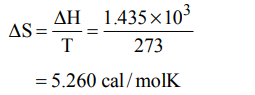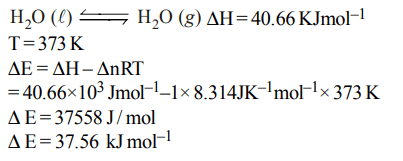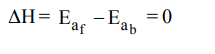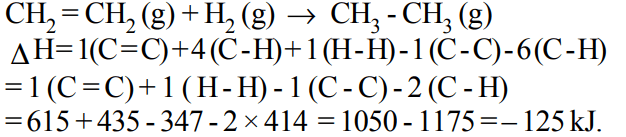## Thermodynamics Questions and Answers Part-9

1. The enthalpy of fusion of water is 1.435 kcal/mol. The molar entropy change for the melting of ice at 0°C is :
a) 10.52 cal / (mol K)
b) 21.04 cal / (mol K)
c) 5.260 cal / (mol K)
d) 0.526 cal / (mol K)

Explanation:2. Standard enthalpy of vapourisation $\triangle_{vap}H^{\circ}$  for water at 100°C is $40.66 k J mol^{-1}$  . The internal energy of vaporisation of water at $100°C\left(kJ mol^{-1}\right)$    is :
a) + 37.56
b) – 43.76
c) + 43.76
d) + 40.66

Explanation:3. Equal volumes of two monoatomic gases, A and B, at same temperature and pressure are mixed. The ratio of specific heats $\left(C_{P}\diagup C_{V}\right)$  of the mixture will be :
a) 0.83
b) 1.50
c) 3.3
d) 1.67

Explanation:4. A reaction having equal energies of activation for forward and reverse reaction has :
a) $\triangle G=0$
b) $\triangle H=0$
c) $\triangle H=\triangle G=\triangle S=0$
d) $\triangle S=0$

Explanation:5. If an endothermic reaction is non-spontaneous at freezing point of water and becomes feasible at its boiling point, then
a) $\triangle H$  is –ve, $\triangle S$  is +ve
b) $\triangle H$  and $\triangle S$  both are +ve
c) $\triangle H$  and $\triangle S$   both are –ve
d) $\triangle H$   is +ve, $\triangle S$   is -ve

Explanation: At low temperature the $\triangle$S is – ve which makes $\triangle$G positive ($\triangle$G = $\triangle$H – T$\triangle$S). But at higher temperature $\triangle$S is +ve which makes the $\triangle$G negative (condition for spontaneity)

6. A heat engine abosrbs heat $Q_{1}$ at temperature $T_{1}$and heat $Q_{2}$ at temperature $T_{2}$. Work done by the engine is $J\left(Q_{1}+Q_{2}\right)$  . This data
a) violates 1st law of thermodynamics
b) violates 1st law of themodynamics if $Q_{1}$ is –ve
c) violates 1st law of thermodynamics of $Q_{2}$ is –ve
d) does not violate 1st law of themodynamics

Explanation: It violate 2nd law of thermodynamics, not 1st

7. For the reactions,
$C+O_{2}\rightarrow CO_{2};\triangle H= -393 J$
$2Zn+O_{2}\rightarrow 2 ZnO;\triangle H= -412 J$
a) carbon can oxidise Zn
b) oxidation of carbon is not feasible
c) oxidation of Zn is not feasible
d) Zn can oxidise carbon

Explanation: $\triangle$H negative shows that the reaction is spontaneous. Higher value for Zn shows that the reaction is more feasible.

8. The internal energy change when a system goes from state A to B is 40 kJ/mole. If the system goes from A to B by a reversible path and returns to state A by an irreversible path what would be the net change in internal energy ?
a) > 40 kJ
b) < 40 kJ
c) Zero
d) 40 kJ

Explanation: For a cyclic process the net change in the internal energy is zero because the change in internal energy does not depend on the path.

9. If at 298 K the bond energies of C — H, C — C, C = C and H — H bonds are respectively 414, 347, 615 and $435 kJ mol^{-1}$ , the value of enthalpy change for the reaction
$H_{2}C=CH_{2}\left(g\right)+H_{2}\left(g\right)\rightarrow H_{3}C-CH_{3}\left(g\right)$
at 298 K will be
a) – 250 kJ
b) + 125 kJ
c) – 125 kJ
d) + 250 kJa) $\left(\triangle S\right)_{V,E}>0,\left(\triangle G\right)_{T,P}<0$
b) $\left(\triangle S\right)_{V,E}=0,\left(\triangle G\right)_{T,P}=0$
c) $\left(\triangle S\right)_{V,E}=0,\left(\triangle G\right)_{T,P}>0$
d) $\left(\triangle S\right)_{V,E}<0,\left(\triangle G\right)_{T,P}<0$
Explanation: For spontaneous reaction, dS > 0 and $\triangle$G and $\triangle$G should be negative i.e. < 0